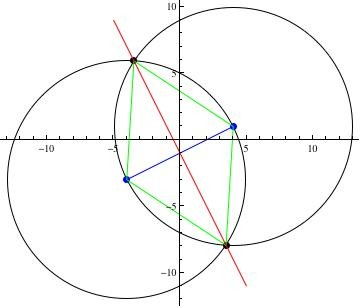# If two vertices of and equilateral triangle are (-4, -3) and (4, 1), find the remaining vertex?

There will be two such vertices. One will be in the second quadrant, and one will be in the fourth quadrant. They will lie on the perpendicular bisector of the line between the given points, and they will be found at the intersection points of circles centered at each point having a radius equal to the distance between the points. (Refer to the figure below.)

The distance between the given points is
r = √((-4-4)^2 + (-3-1)^2) = √(64+16) = √80

The circle centered at (-4, -3) has equation
(x + 4)^2 + (y + 3)^2 = 80
x^2 + 8x + 16 + y^2 + 6y + 9 = 80    (expansion of the equation. Call this )

The circle centered at (4, 1) has equation
(x - 4)^2 + (y - 1)^2 = 80
x^2 - 8x + 16 + y^2 - 2y + 1 = 80    (expansion of the equation. Call this )

We can set  =  and solve for y.
x^2 + 8x + 16 + y^2 + 6y + 9 = 80 = x^2 - 8x + 16 + y^2 - 2y + 1
8x + 6y + 9 = -8x - 2y + 1    (subtract x^2+16+y^2 from both sides)
6y = -16x - 2y - 8    (subtract 8x+9 from both sides)
8y = -16x - 8    (add 2y)
y = -2x - 1    (divide by 8. This is the equation of the red line. Call this )

We can substitute  into  and solve for x. In the figure, this gives the x coordinate of the intersection points of the red line and the lower circle.
x^2 + 8x + 16 + (-2x - 1)^2 + 6(-2x - 1) + 9 = 80
x^2 + 8x + 16 + 4x^2 + 4x + 1 - 12x - 6 + 9 = 80    (eliminate parentheses)
5x^2 + 20 = 80    (collect terms)
5x^2 = 60    (subtract 20)
x^2 = 12    (divide by 5)
x = ±2√3    (take the square root)

We can use this result in  to find the corresponding values of y. (Positive sign first.)
y = -2(2√3) - 1 = -4√(3) - 1
The quadrant IV vertex is (2√3, -4√(3) - 1).

y = -2(-2√3) - 1 = 4√(3) - 1
The quadrant II vertex is (-2√3, 4√(3) - 1).thanked the writer.
Mayett Alunsagay commented
ThanK You so Much!!God Bless!!
Mark Mottian commented
I ask yet again, where do you get all the funky diagrams?
Oddman commented
Mark, Yet again, I will tell you that I draw them. I use the plotting and graphics primitives available in Mathematica, which is a benefit of my job. It often means I have to solve the problem different ways three or four times to get everything placed properly. Labeling is harder, so you don't see much of that.